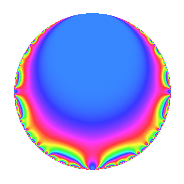# Properties

 Label 39.1.dLevel 39 Weight 1 Character orbit d Rep. character $$\chi_{39}(38,\cdot)$$ Character field $$\Q$$ Dimension 1 Newforms 1 Sturm bound 4 Trace bound 0

# Related objects

## Defining parameters

 Level: $$N$$ = $$39 = 3 \cdot 13$$ Weight: $$k$$ = $$1$$ Character orbit: $$[\chi]$$ = 39.d (of order $$2$$ and degree $$1$$) Character conductor: $$\operatorname{cond}(\chi)$$ = $$39$$ Character field: $$\Q$$ Newforms: $$1$$ Sturm bound: $$4$$ Trace bound: $$0$$

## Dimensions

The following table gives the dimensions of various subspaces of $$M_{1}(39, [\chi])$$.

Total New Old
Modular forms 3 3 0
Cusp forms 1 1 0
Eisenstein series 2 2 0

The following table gives the dimensions of subspaces with specified projective image type.

$$D_n$$ $$A_4$$ $$S_4$$ $$A_5$$
Dimension 1 0 0 0

## Trace form

 $$q - q^{3} - q^{4} + q^{9} + O(q^{10})$$ $$q - q^{3} - q^{4} + q^{9} + q^{12} - q^{13} + q^{16} - q^{25} - q^{27} - q^{36} + q^{39} + 2q^{43} - q^{48} + q^{49} + q^{52} - 2q^{61} - q^{64} + q^{75} - 2q^{79} + q^{81} + O(q^{100})$$

## Decomposition of $$S_{1}^{\mathrm{new}}(39, [\chi])$$ into irreducible Hecke orbits

Label Dim. $$A$$ Field Image CM RM Traces $q$-expansion
$$a_2$$ $$a_3$$ $$a_5$$ $$a_7$$
39.1.d.a $$1$$ $$0.019$$ $$\Q$$ $$D_{2}$$ $$\Q(\sqrt{-3})$$, $$\Q(\sqrt{-39})$$ $$\Q(\sqrt{13})$$ $$0$$ $$-1$$ $$0$$ $$0$$ $$q-q^{3}-q^{4}+q^{9}+q^{12}-q^{13}+q^{16}+\cdots$$